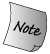### 6.4. 语句作用域

Some statements permit variable definitions within their control structure:

```     while (int i = get_num())
cout << i << endl;
i = 0; // error: i is not accessible outside the loop
```Variables defined in a condition must be initialized. The value tested by the condition is the value of the initialized object. 在条件表达式中定义的变量必须初始化，该条件检验的就是初始化对象的值。

Variables defined as part of the control structure of a statement are visible only until the end of the statement in which they are defined. The scope of such variables is limited to the statement body. Often the statement body itself is a block, which in turn may contain other blocks. A name introduced in a control structure is local to the statement and the scopes nested inside the statement:

```     // index is visible only within the for statement
for (vector<int>::size_type index = 0;
index != vec.size(); ++index)
{ // new scope, nested within the scope of this for statement
int square = 0;
if (index % 2)                      // ok: index is in scope
square = index * index;
vec[index] = square;
}
if (index != vec.size()) // error: index is not visible here
```

If the program needs to access the value of a variable used in the control statement, then that variable must be defined outside the control structure:

```     vector<int>::size_type index = 0;
for ( /* empty */ ; index != vec.size(); ++index)
// as before
if  (index != vec.size()) // ok: now index is in scope
// as before
```Earlier versions of C++ treated the scope of variables defined inside a for differently: Variables defined in the for header were treated as if they were defined just before the for. Older C++ programs may have code that relies on being able to access these control variables outside the scope of the for. 早期的 C++ 版本以不同的方式处理 for 语句中定义的变量的作用域：将 for 语句头定义的变量视为在 for 语句之前定义。有些更旧式的 C++ 程序代码允许在 for 语句作用域外访问控制变量。

One advantage of limiting the scope of variables defined within a control statement to that statement is that the names of such variables can be reused without worrying about whether their current value is correct at each use. If the name is not in scope, then it is impossible to use that name with an incorrect, leftover value.